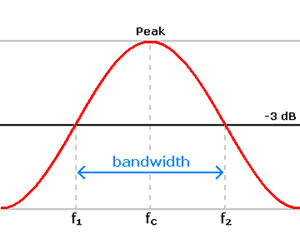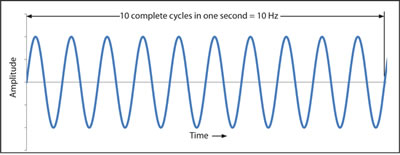# Difference between Bandwidth and Frequency

##### Key Difference: Bandwidth has two major definitions – one in computing and the other in signal processing. On the other hand, frequency is the number of complete cycles per second in alternating current direction.Bandwidth and frequency are two concepts that are common for science and engineering majors around the world. These can also be commonly be found in computing. While, these may seem similar, but they differ each other in many ways.

Bandwidth has two major definitions – one in computing and the other in signal processing. In terms of computing bandwidth refers to the rate at which data can transfer. This basically means the amount of data that can be transferred from one location to another location in a given timeframe, usually expressed in bits per second. Modern network bandwidths typically have speeds that are measured in millions of bits per second, better recognized as Mbps.

However note that bandwidth is not the only factor that affects the performance of a network. Other factors also include packet loss, latency and jitter, all of which degrade network throughput and make a link perform like one with lower bandwidth.

The second definition, commonly used in signal processing, is the range of frequencies an electronic signal uses on a given transmission medium. This means that the term bandwidth refers to difference between the highest-frequency signal component and the lowest-frequency signal component. It is measured in Hertz per second and is the first and original definition of bandwidth before the introduction of this word into computers.On the other hand, frequency is the number of complete cycles per second in alternating current direction. Frequency is used for oscillating or varying currents. The number of cycles completed are used to determine the speed. For example, if a current completes 1 cycle in 1 second, then the speed would be 1 Hertz or Hz. If the current completes 60 cycles in 1 second, it would be 60Hz. The clock speed of a computer is usually measured in megahertz (MHz) or gigahertz (GHz).

Frequency also plays an important in wireless communication, where the frequency of a signal is mathematically related to the wavelength.

Comparison between Bandwidth and Frequency:

 Bandwidth Frequency Definition The rate at which data is transferred from one network to another The difference between the highest frequency signal component and the lowest-frequency signal component The number of complete cycles per second in alternating current direction Time measurement Per second Per second Used in Computing, signal processing Science and engineering to specify the rate of oscillatory and vibratory phenomena, such as mechanical vibrations, audio (sound) signals, radio waves, and light Standard Unit Hertz; Hz Hertz; Hz Larger Units Kilohertz, megahertz, gigahertz, terahertz Kilohertz, megahertz, gigahertz, terahertz

Image Courtesy: commons.wikimedia.org, minelab.com